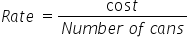Mathematics
Easy

Question

# Nathan paid \$56.70 for 70 cans of juice. It would cost him ___________, if he were to buy 3 cans at the same rate.

## 2.53.12 2.43      2.7Hint:

## The correct answer is: 2.43

### Cost of 70 cans of juices is \$56.70We have to find the rate of per can. We will find it by taking the ratio of the cost of cans and number of cans.. As the rate is constant, we will use this value to find cost of 3 cansSo the equation becomesSo the rate = \$0.81 per canLet’s find the cost of 3 cansWe will rearrange this for cost.For this we will multiply both sides by number of cans.Rate × Number of cans = CostCost = Rate × Number of cansSubstituting the values we have foundCost = 0.81 × 3Cost = 2.43Therefore, cost of 3 cans of juices is \$2.43.

We should be careful about the units. We should make sure that the units of same quantities are same.

### Related Questions to study#### With Turito Foundation.#### Get an Expert Advice From Turito.